Courses

# RS Aggarwal Solutions: Whole Numbers Exercise - 3F Notes | EduRev

## Class 6 : RS Aggarwal Solutions: Whole Numbers Exercise - 3F Notes | EduRev

The document RS Aggarwal Solutions: Whole Numbers Exercise - 3F Notes | EduRev is a part of the Class 6 Course Mathematics (Maths) Class 6.
All you need of Class 6 at this link: Class 6

Objective questions
Mark (✔) against the correct answer in each of the following :
Q.1. The smallest whole number is
(a) 1
(b) 0
(c) 2
(d) none of these
Ans. The smallest whole number is 0

Q.2. The least number of 4 digits which is exactly divisible by 9 is
(a) 1018
(b) 1026
(c) 1009
(d) 1008
Ans. The least 4-digit number = 1000
On dividing 1000 by 9, we get
Remainder = 1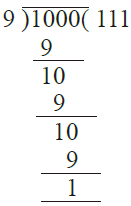∴ Least 4-digit number which is
Divisible by 9 = 1000 – 1 + 9
= 1000 + 8 = 1008

Q.3. The largest number of 6 digits which is exactly divisible by 16 is
(a) 999980
(b) 999982
(c) 999984
(d) 999964
Ans.
The largest 6-digit number = 999999
On dividing by 16, we get
Remainder = 15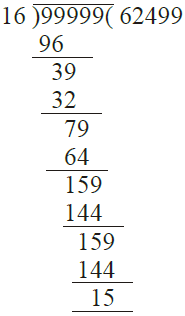The greatest 6-digit number divisible by 16
= 999999 – 15 = 999984

Q.4. What least number should be subtracted from 10004 to get a number exactly divisible by 12 ?
(a) 4
(b) 6
(c) 8
(d) 20
Ans.
On dividing 10004 by 12, we get
remainder = 8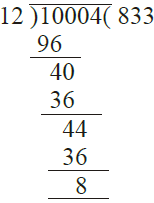∴ 8 is to be subtracted from 10004

Q.5. What least number should be added to 10056 to get a number exactly divisible by 23 ?
(a) 5
(b) 18
(c) 13
(d) 10
Ans.
On dividing 10056 by 23
We get remainder = 12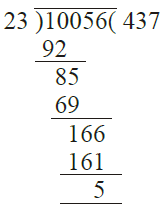∴ The least number to be added
= 23 – 5 = 18

Q.6. What whole number is nearest to 457 which is divisible by 11 ?
(a) 450
(b) 451
(c) 460

(d) 462
Ans.
On dividing 457 by 11
We get remainder = 6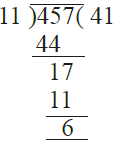Which is greater than half of 11
∴ The number nearest to 457 which is divisible 11 will be = 457 – 6 + 11 = 457 + 5 = 462

Q.7. How many whole numbers are there between 1018 and 1203 ?
(a) 185
(b) 186
(c) 184
(d) none of these
Ans. Whole number between 1018 and 1203 are 1019 to 1202 are 1202 – 1018

= 184

Q.8. A number when divided by 46 gives 11 as quotient and 15 as remainder. The number is
(a) 491
(b) 521
(c) 701
(d) 679
Ans. Divisor = 46
Quotient = 11
Remainder = 15
∴ Number = Divisor × Quotient + Remainder
= 46 × 11 + 15 = 506 + 15 = 521

Q.9. In a division sum, we have dividend = 199, quotient = 16 and remainder = 7. The divisor is
(a) 11
(b) 23
(c) 12
(d) none of these
Ans.
Dividend = 199
Quotient = 16
Remainder = 7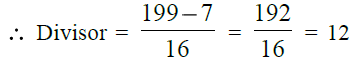Q.10. 7589 – ? = 3434
(a) 11023
(b) 4245
(c) 4155
(d) none of these
Ans.
7589 – ? = 3434

∴ Required number = 7589 – 3434
= 4155

Q.11. 587 × 99 = ?
(a) 57213
(b) 58513
(c) 58113
(d) 56413
Ans. 587 × 99 = 587 × (100 – 1)
= 587 × 100 – 587 × 1
= 58700 – 587 = 58113

Q.12. 4 × 538 × 25 = ?
(a) 32280
(b) 26900
(c) 53800
(d) 10760
Ans.
4 × 538 × 25 = 538 × 4 × 25
= 538 × 100 = 53800

Q.13. 24679 × 92 + 24679 × 8 = ?
(a) 493580
(b) 1233950
(c) 2467900
(d) none of these
Ans. 24679 × 92 + 24679 × 8
= 24679 × (92 + 8)
= 24679 × 100 = 2467900

Q.14. 1625 × 1625 – 1625 × 625 = ?
(a) 1625000
(b) 162500
(c) 325000
(d) 812500
Ans.
1625 × 1625 – 1625 × 625
= 1625 (1625 – 625) = 1625 × 1000
= 1625000

Q.15. 1568 × 185 – 1568 × 85 = ?
(a) 7840
(b) 15680
(c) 156800
(d) none of these
Ans.
1568 × 185 – 1568 × 85
= 1568 (185 – 85) = 1568 × 100
= 156800

Q.16. (888 + 777 + 555) = (111 × ?)
(a) 120
(b) 280
(c) 20
(d) 140
Ans. 888 + 777 + 555 = 111 × ?
= 11 (8 + 7 + 5) = 111 × 20

Q.17. The sum of two odd numbers is
(a) an odd number
(b) an even number
(c) a prime number
(d) a multiple of 3
Ans.
The sum of two odd number is an even number

18. The product of two odd numbers is
(a) an odd number
(b) an even number
(c) a prime number

(d) none of these
Ans. The product of two odd numbers is an odd number

Q.19. If a is a whole number such that a + a = a, then a = ?
(a) 1
(b) 2
(c) 3
(d) none of these
Ans. If a is a whole number such that
a + a = a, then a = 0
as 0 + 0 = 0
∴ None of these

Q.20. the predecessor of 10000 is

(a) 10001
(b) 9999
(c) none of these
Ans. The predecessor of 10000 is
10000 – 1 = 9999

Q.21. The successor of 1001 is
(a) 1000
(b) 1002
(c) none of these
Ans. The successor of 1001 is 1001 + 1 1002

Q.22. The smallest even whole number is

(a) 0
(b) 2
(c) none of these
Ans. The smallest even whole number is 2

Offer running on EduRev: Apply code STAYHOME200 to get INR 200 off on our premium plan EduRev Infinity!

## Mathematics (Maths) Class 6

191 videos|221 docs|43 tests

,

,

,

,

,

,

,

,

,

,

,

,

,

,

,

,

,

,

,

,

,

;